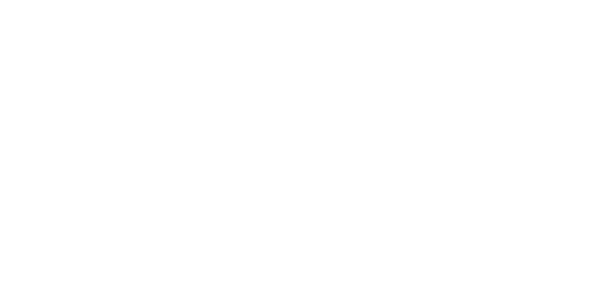VBA关于日期时间的处理

提取日期里的年月日

'获取年
year=Year(Range("A1"))
'获取月
month=Month(Range("A1"))
'获取日
day=Day(Range("A1"))

日期之间的运算

两个日期之间的差

DateDiff(interval, date1, date2 [,firstdayofweek[, firstweekofyear]])

Interval - 一个必需的参数。 它可以采用以下值。

• d - 一年中的一天
• m - 一年中的月份
• y - 一年中的年份
• yyyy - 年份
• w - 工作日
• ww - 星期
• q - 季度
• h - 小时
• m - 分钟
• s - 秒钟

'天数的差异
DateDiff("d",range("A1"),Range("B1"))
'月份的差异
DateDiff("m",range("A1"),Range("B1"))
'年份的差异
DateDiff("y",range("A1"),Range("B1"))

日期月份的增加

1. Edate() 是与指定日期 (start_date) 相隔（之前或之后）指示的月份数。 使用函数 EDATE 可以计算与发行日处于一月中同一天的到期日的日期。

vb EDATE(start_date, months) '如果计算2021/1/31后面的一个月的日期,这里会得到2021/2/28 EDATE("2021/1/31",1) ¨G2G vb EOMONTH(start_date, months) '2021/1/1后面一个月的最后一天，及2021/2/28 EOMONTH("2021/1/1", 1) ¨G3G vb Sub GETDATE() '设定循环 For i = 0 To 11 '起始月份 dd = "2021/1/1" 'VBA调用EXCEL函数EoMonth newdate = WorksheetFunction.EoMonth(dd, i) ¨K13K End SubCatfish(鲶鱼) CMS V 6.4.0